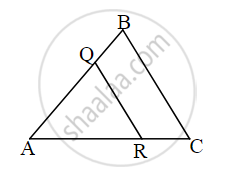# Choose the correct alternative: ∆ABC ∼ ∆AQR. ABAQ=75, then which of the following option is true? - Geometry Mathematics 2

MCQ

Choose the correct alternative:

∆ABC ∼ ∆AQR. "AB"/"AQ" = 7/5, then which of the following option is true?

• A–Q–B

• A–B–Q

• A-C–B

• A–R–B

#### Solution

A–Q–BΔABC ∼ ΔAQR and "AB"/"AQ" = 7/5

⇒ ΔABC is greater and ΔAQR is smaller.

∠A is a common angle.

∴ We get, A-Q-B

Is there an error in this question or solution?
Chapter 4: Geometric Constructions - Q.1 (A)
Share